A good grasp on Excel functions can enhance your ability to perform day to day business tasks. Although there are hundreds of formulas in Microsoft Excel’s repertoire, most users only ever use a handful of them and you probably won’t ever need to use most of them. We handpicked some of the crafty and useful functions Excel has to offer and created this guide. Let’s take a look at some of the most commonly used functions together. You can download our sample workbook here.

## VLOOKUP, HLOOKUP

VLOOKUP is one of the most famous functions of Excel as it allows matching data from a table to user selection. Although not as widely known, HLOOKUP does the same thing, although in a transposed search pattern.

Both functions look up and find a value within a table. While VLOOKUP uses the first column to search for the lookup value (first argument of both functions); HLOOKUP uses first row. After finding lookup value in search column or row, both function return the value in specified column or row number in the table array selection. The last parameter determines whether the formula should look for an exact or approximate string (range lookup).

The syntax for these two formulas are,

VLOOKUP(Lookup_value, table_array, col_index_num, [range_lookup])

HLOOKUP(Lookup_value, table_array, row_index_num, [range_lookup])

### Sample use

Let’s assume that we need to find which department a user belongs to. Using a VLOOKUP formula and targeting the appropriate fields, we can create a search engine. Typing in “HALEY BATES” will find the corresponding column (B9) in our table (A1:C6) and will return the value of the Department column (3) and will look for the exact value entered (FALSE).## IF, AND, OR

IF function is a handy and easy to use function. You can have Excel check for a logical test and set a value to the cell or run another formula, based on the result of the test, which can be TRUE or FALSE.

The syntax for this formula is,

IF(logical_test, value_if_true, [value_if_false])

### Sample Use

Any ‘this or that’ situation can be given as an example. Here, we created a simple list of grades and are trying to find whether students passed or failed the test. If the grade is equal to or greater than 70, it’s a pass, otherwise a fail (B2>=70). We can also have Excel print “Pass” if this statement is true, if not then formula will return “Fail”.To enhanced the capabilities of an IF function, it is often combined with other logical functions. Most popular functions include AND and OR functions. AND and OR functions compare their arguments  and give TRUE or FALSE as a result. These return values can be used in an IF formula logical test argument to test more than one condition at the same time.

The syntax for these two formulas are,

AND(logical1, [logical2], ...)

OR(logical1, [logical2], ...)

In an alternative scenario, let's assume that everyone has a total of 3 grades (exams and final test). “Pass” condition requires two options to be evaluated,

1. All grades should be equal to or greater than 60
2. Average of grades should be equal to or greater than 70

"All grades should be equal or greater than 60” condition requires “all” grades to be equal to or greater than 60. We can use AND function to combine these statements,

AND(B7>=60,C7>=60,D7>=60)

On the other hand; if one of the two options is enough for a passing grade, we can use the OR function to compare the two,

OR(AND(B7>=60,C7>=60,D7>=60),AVERAGE(B7:D7)>=70)## NOW, TODAY

NOW and TODAY functions return the “computer’s current time and date” respectively. We must stress the “computer’s” part, because the formula will return whatever your computer thinks the time is, whether it’s right or not. Both formulas are extremely easy to use and they don’t use any arguments, you simply type them in with the open and closed parentheses.

The syntax for these two formulas are,

=NOW()

=TODAY()

## SUM

The SUM function, as the name suggests, adds things. It adds all numerical values defined in its arguments. Arguments can be a static numerical value, a single cell, or range. You can add up to 255 arguments into a single SUM function.

The syntax is,

SUM(number1,[number2],...)

### Sample Use

Summary of

• 2 single cells: B2, B3
• 2 ranges: B4:B6, B7:C10
• Static value: 14

Please note that the non-numeric value in C8 (“a” character) is ignored.## AVERAGE

AVREAGE function is used exactly the same way as the SUM function. It is used to calculate the average of all numerical values in the syntax. Static values, single cells, and ranges can be used as arguments and argument limit is 255 like in SUM function.

The syntax is,

AVERAGE(number1,[number2],...)

### Sample Use

Summary of

• 2 single cells: B2, B3
• 2 ranges: B4:B6, B7:C10
• Static value: 14

Please note that the non-numeric value in C8 (“a” character) is ignored.## SUMIF, COUNTIF

SUMIF and COUNTIF functions add up or count the number of values, only when a given criteria is met. Both functions search the range of arguments and check the condition stated in the criteria arguments. SUMIF function also has an optional argument named sum_range that can be used if the sum values and search values are in different ranges.

Criteria argument can be set for logical conditions like equal, not equal, greater than, equal to or greater than, lesser than, and equal to or lesser than. For the equal to condition; you can set a static value or a cell reference and for others, you can use operators. For example, to search for values “greater than value of C5 cell” the criteria parameter should be “>”&C5.

The syntax for these two formulas are,

COUNTIF(range, criteria)

SUMIF(range, criteria, [sum_range])

### Sample Use - COUNTIF

Let’s use the same table we used in the previous example IF, OR, AND.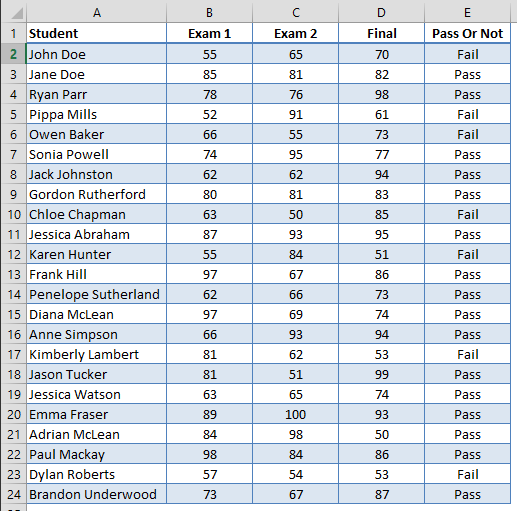To find the number of students who passed, we can make a search on the Pass Or Not column, and count the “Pass” strings. The formula should be,

=COUNTIF(\$E\$2:\$E\$24,"Pass")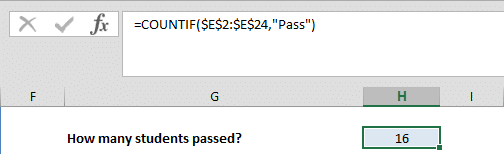To find the number of students who got at least 70 in the Final,

=COUNTIF(\$D\$2:\$D\$24,">="&70)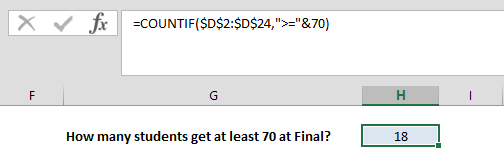### Sample Use - SUMIF

For this example, we’re going to use a table of Property Values and Commission rates. Assume that we need to calculate the total commissions for property valued over \$160,000. The formula syntax for this case would be,

=SUMIF(A27:A30,">160000",B27:B30)## RIGHT, LEFT, MID

These three are the fundamental text functions of Excel and are used the grab certain parts from texts. All functions use text as the first argument. While RIGHT and LEFT functions have one more argument to determine the number of characters (num_bytes) to be included, MID function uses two more arguments to determine where the characters (start_num) start and the number of characters (num_bytes) to be extracted.

As the names suggest, LEFT, RIGHT and MID grab text form left, right and the middle respectively.

The syntax for these formulas are,

LEFT(text, [num_chars])

RIGHT(text, [num_chars])

MID(text, start_num, num_chars)

### Sample use

The cell B5 contains the text we want to extract. Here are some examples for the use of these formulas,

• 3 characters from left: =LEFT(\$B\$1,3)
• 5 characters from right =RIGHT(\$B\$1,5)
• Characters from 4 to 10 =MID(\$B\$1,4,7)

Please note that finding characters between 4th and 10th requires setting start_num 4 and num_chars 7.  num_chars is not the last character’s index, but it’s how many characters are needed to reach that point.## ROUND

The ROUND function allows you to manage the decimal portion of a numeric value. It takes 2 arguments. The first one is the numeric value itself (number). This can be a static number or a cell reference as usual. The second argument is the number of decimals to round to (num_digits).

The syntax is,

ROUND(number, num_digits)

### Sample Use

Rounding 14.375 to 2 digits will result 14.38. If we use 1 as num_digits argument, the result will be 14.4.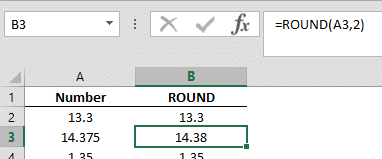Alternatively; negative numbers can be used as num_digits to round to the left of the decimal point. For example, if num_digit is -3, this means 1000 which is 3 power of 10.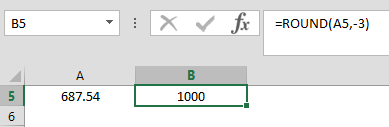There are hundreds and hundreds of formulas in Excel but we can’t cover each and every one of them. But don’t stop here! Keep expanding your knowledge and practice with formulas to master Excel. You will feel the difference in your productivity in no time.